# AP Calculus AB Practice Test 41

### Test Information10 questions20 minutes

Calculator Disallowed

1.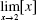(where [x] is the greatest integer in x) is

2.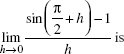3. If f (x) = x ln x, then f(e) equals

4. The equation of the tangent to the curve 2x2 - y4 = 1 at the point (-1, 1) is

5. On which interval(s) does the function f (x) = x4 - 4x3 + 4x2 + 6 increase?

6.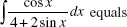7. A relative maximum value of the function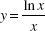is

8. If a particle moves on a line according to the law s = t5 + 2t3, then the number of times it reverses direction is

9.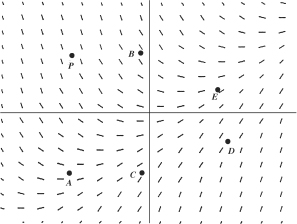A particular solution of the differential equation whose slope field is shown abovecontains point P. This solution may also contain which other point?

10. Let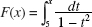. Which of the following statements is (are) true?

I. The domain of F is x ≠ ±1.

II. F(2) > 0.

III. The graph of F is concave upward.